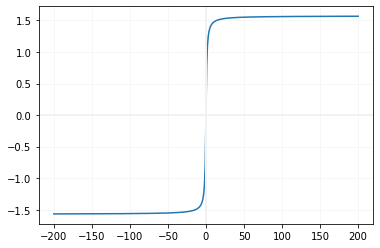# atan()

atan(x) returns arc tan of x. Input number is in radian
``````import math
print(math.atan(0))      # 0.0
print(math.atan(0.1))    # 0.09966865249116204
print(math.atan(0.5))    # 0.4636476090008061
print(math.atan(1.5))    # 0.982793723247329
print(math.atan(10))     # 1.4711276743037347
print(math.atan(50))     # 1.550798992821746
print(math.atan(500))    # 1.5687963294615568``````
Note that all the inputs are in radian.
Using negative input value
``````import math
print(math.atan(-0.1))    # -0.09966865249116204
print(math.atan(-0.5))    # -0.4636476090008061
print(math.atan(-1.5))    # -0.982793723247329
print(math.atan(-10))     # -1.4711276743037347
print(math.atan(-50))     # -1.550798992821746
print(math.atan(-500))    # -1.5687963294615568``````

## Inputs in degree

We can convert radian value to degree and use the same
``````import math
in_degree = 57
print(math.atan(in_redian))``````
Output
``0.782810326348054``

## Drawing graph of atan()

In our Trigonometric language atan is also know as arctan. Using this we will use Matplotlib to generate graph of atan``````import matplotlib.pyplot as plt
x=[]
y=[]
i=-1
while (i<=1):
x.append(i)
y.append(math.atan(i))
i=i+0.01
plt.plot(x,y)
plt.show()``````

Subscribe to our YouTube Channel here

## Subscribe

* indicates required
Subscribe to plus2netplus2net.com